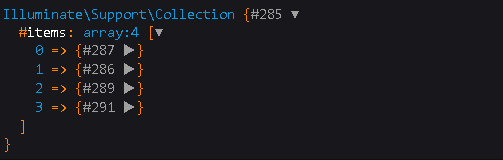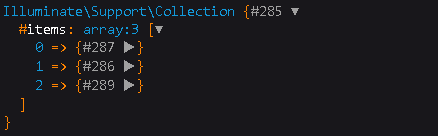# Elevate Your Laravel Skills: Dive into whereNull & whereNotNull Queries

0

## How to Use Laravel Eloquent whereNull and whereNotNull methodIn this article we will cover how can you use whereNull and whereNotNull in Eloquent Laravel . We will see how to use these methods with example and also different ways to use these methods .

whereNull method is basically used to filter out the rows having a specified field or column will NULL value . This method basically takes one parameter that is the ' column_name ' .

Similarly whereNotNull method is used to filter out the rows having a specified field or column with no NULL value . This method is also takes only one parameter that is ' column_name ' .

To understand these methods we take the example of following table to understand our queries .

### Laravel Eloquent whereNull :

#### SQL Query :

`SELECT * FROM products`
```
WHERE created_at IS NULL;
```

#### Laravel Syntax 1:

```\$data=Model_Name::select('*')

->whereNull('column_name')

->get();

dd(\$data);
```

#### Laravel Example 1:

```\$data=Product::select('*')

->whereNull('created_at')

->get();

dd(\$data);
```
```
```

#### Laravel Syntax 2:

```\$data=DB::table('table_name')

->whereNull('column_name')

->get();

dd(\$data);
```

#### Laravel Example 2:

```\$data=DB::table('products')

->whereNull('created_at')

->get();

dd(\$data);
```

### Output :### Laravel Eloquent whereNotNull :

#### SQL Query :

`SELECT * FROM products`
`WHERE created_at IS NOT NULL;`

#### Laravel Syntax 1:

```\$data=Model_Name::select('*')

->whereNotNull('column_name')

->get();

dd(\$data);
```

#### Laravel Example 1:

```\$data=Product::select('*')

->whereNotNull('created_at')

->get();

dd(\$data);
```

#### Laravel Syntax 2:

```\$data=DB::table('table_name')

->whereNotNull('column_name')

->get();

dd(\$data);
```

#### Laravel Example 2:

```\$data=DB::table('products')

->whereNotNull('created_at')

->get();

dd(\$data);```

### Output :These are the different ways to use Laravel Eloquent whereNull and whereNotNull method . Hope it helped you .